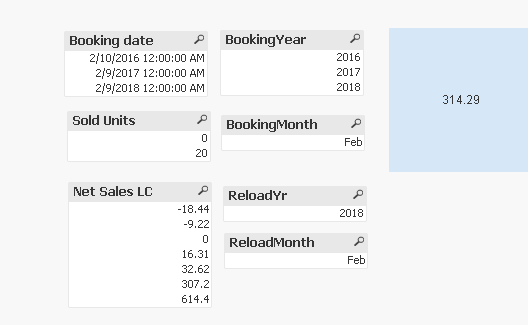New to QlikView

Discussion board where members can get started with QlikView.

New Contributor II

Sum(if(and formula

Hi,

This is driving me crazy. I'm trying to summarize all bookings that has the same month and year as the reload time with this formula;

Sum(if(year([Booking date])=year(ReloadTime()) and month([Booking date])=month(ReloadTime()),[Net Sales LC]))

However it returns 0.

Can anyone help me?

Best,

Simon

1 Solution

Accepted Solutions
Highlighted
Esteemed Contributor III

Re: Sum(if(and formula

Can you try this and let me know what you getting?.

SumSales:

LOAD *, Year([Booking date]) AS BookingYear, Month([Booking date]) AS BookingMonth,

[Sold Units],

[Net Sales LC]

FROM

(ooxml, embedded labels, table is page);

Change the excel path accordingly and run. By the way your data for booking date has January and when you do reloadtime which is going to be feb (which is current month and year) you will get all 0's. So i changed the Booking date values to Feb and ran the expression.

This is what i see when i run the expression.

= Sum(if(BookingYear = ReloadYr AND BookingMonth = ReloadMonth,[Net Sales LC]))8 Replies
Esteemed Contributor III

Re: Sum(if(and formula

Can you share some sample to look into?

New Contributor II

Re: Sum(if(and formula

Hi,

Yes, a very small sample attached.

Best,

SimonMVP

Re: Sum(if(and formula

At first look, I see bracket issues:

Sum(if(year([Booking date])=year(ReloadTime()) and month([Booking date])=month(ReloadTime()),[Net Sales LC]))

If this doesn't help, try to share more information about your issue and share a sample/screen shot may be.

Honored Contributor

Re: Sum(if(and formula

Please share sample data

New Contributor II

Re: Sum(if(and formula

Attached is a small sample

Highlighted
Esteemed Contributor III

Re: Sum(if(and formula

Can you try this and let me know what you getting?.

SumSales:

LOAD *, Year([Booking date]) AS BookingYear, Month([Booking date]) AS BookingMonth,

[Sold Units],

[Net Sales LC]

FROM

(ooxml, embedded labels, table is page);

Change the excel path accordingly and run. By the way your data for booking date has January and when you do reloadtime which is going to be feb (which is current month and year) you will get all 0's. So i changed the Booking date values to Feb and ran the expression.

This is what i see when i run the expression.

= Sum(if(BookingYear = ReloadYr AND BookingMonth = ReloadMonth,[Net Sales LC]))MVP

Re: Sum(if(and formula

Hi Simon,

Your expression looks good to me. There are 2 possibilities.

1)  [Booking date] field might be loaded as text format in Qlikview. So the year and month function returns nothing and your expression returns 0. So check the format of the [Booking Date] field and change it to date format (If it is not loaded as date format).

2) If you reload your application on Feb month and if there are no sales for the month of Feb then the net sales will be 0. So check whether is there any sales for the month of Feb or not.

Good luck.

New Contributor II

Re: Sum(if(and formula

Thanks - that worked! I also expanded it to weeks